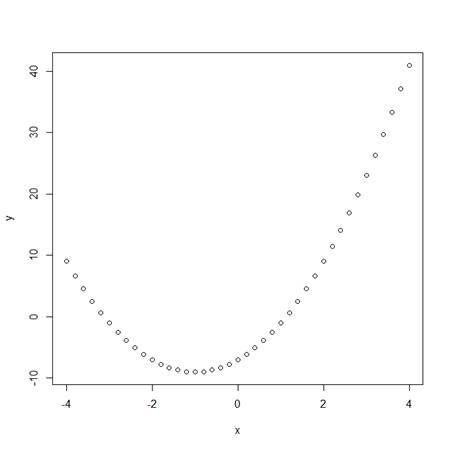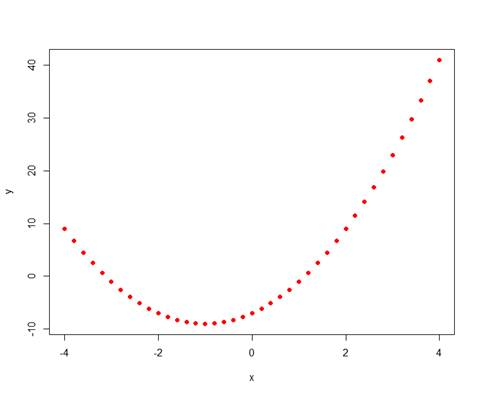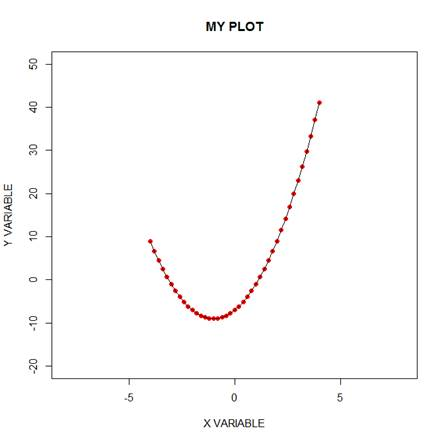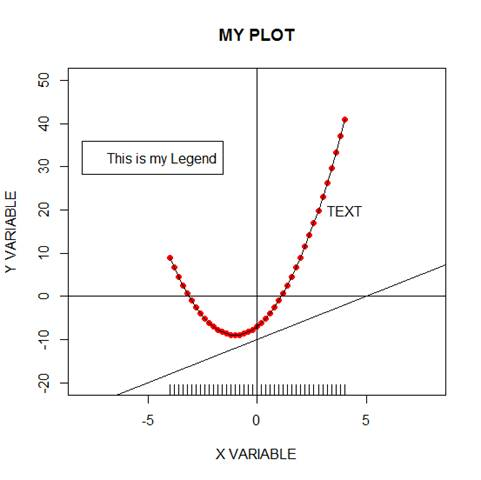# R Is Not So Hard! A Tutorial, Part 6: Basic Plotting in RIn Part 6, let’s look at basic plotting in R.  Try entering the following three commands together (the semi-colon allows you to place several commands on the same line).

`x <- seq(-4, 4, 0.2) ;  y <- 2*x^2 + 4*x - 7`
`plot(x, y)`This is a very basic plot, but we can do much better. Let’s build a nicer plot in several steps.

`plot(x, y, pch = 16, col = "red")`The argument pch = 16 gave us solid circles, while col = “red” coloured those circles red.

Now try:

`plot(x, y, pch = 16, col = "red", xlim = c(-8, 8), ylim = c(-20, 50),`
`main = "MY PLOT", xlab = "X VARIABLE" , ylab = "Y VARIABLE")`

You can see what the main argument and the xlim and ylim arguments achieved. Now try:

`plot(x, y, pch = 16, col = "red", xlim = c(-8, 8), ylim = c(-20, 50),`
`main = "MY PLOT", xlab = "X VARIABLE" , ylab = "Y VARIABLE")`
`lines(x, y)`Note that the lines command is used after the plot command. Now enter the following syntax.

`abline(h = 0)`
`abline(v = 0)`
`abline(-10, 2)` # Draws a line of intercept -10 and slope 2.
`text(4, 20, "TEXT")` # Now your text is located at the point (4, 20) on your plot.

`legend(-8,36,"This is my Legend")`

Your legend is now centered on the point (-4, 36)

`rug(x)`

The rug command indicates the location of points on the horizontal axis. Here is the final plot:That wasn’t so hard! In blog 7 we will look at some more sophisticated plotting techniques in R.